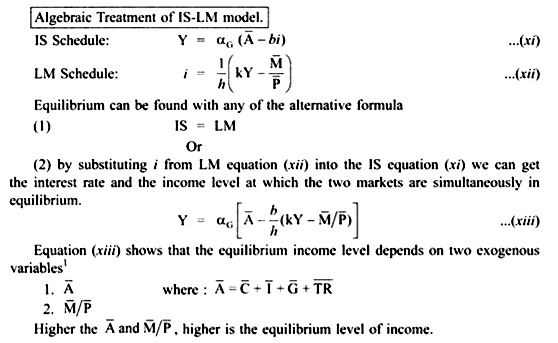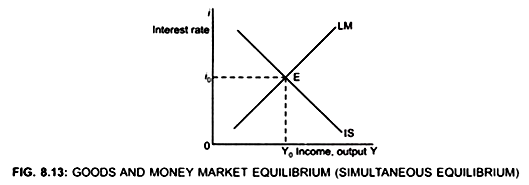# Goods and Money Market Equilibrium (With Diagram)

The IS-LM model finds the value of income and interest rate which simultaneously clears the goods and money market.

The interest rate and the income level should be such that both the markets are in equilibrium. The IS-LM shows the interaction between the goods and the money market.

The model finds the value of income and the interest rate which simultaneously clears the goods and the money market. It appears that the equilibrium income level cannot be determined without first knowing the rate of interest and the interest rate cannot be determined without first knowing the income level.

Therefore, a simultaneous solution is required to find the equilibrium level of income and the interest rate. This is done through the Hicksian IS-LM framework.Although LM curve shows several interest rate consistent with monetary equilibrium and IS curve shows several interest rate consistent with product market equilibrium but there is only one income level and interest rate at which both product and money market is in equilibrium. This is at point E. Since at point E (Fig. 8.13).

(i) Actual expenditure = Planned expenditure

Or

I = S

and (ii) Demand for real balance = Supply of real balance,